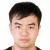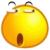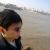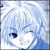PHP 两个数组取交集问题

【推荐阅读】滴滴出行的数据架构和信息流处理思路解析->>>\$a= array('a','c');

\$b = array('a'=>1,'b'=>2,'c'=>3,'d'=>4,'e'=>5);

0array_intersect_key();  用这个方法，使用键名计算数组的交集

0array_intersect_key();  用这个方法，使用键名计算数组的交集

\$res = [];
foreach (\$a as \$k)
{
isset(\$b[\$k]) && ! isset(\$res[\$k]) && \$res[\$k] = \$b[\$k];
}
var_export(\$res);
0\$c=array_intersect_key(\$b,\$a) 可以得到结果..\$a数组的key是0，1，\$b的key是a,b,c，这个函数明显不适合
0
d
array_intersect_key(\$b,array_flip(\$a));